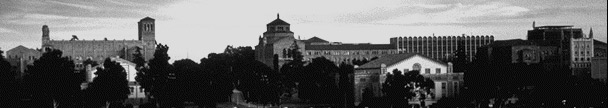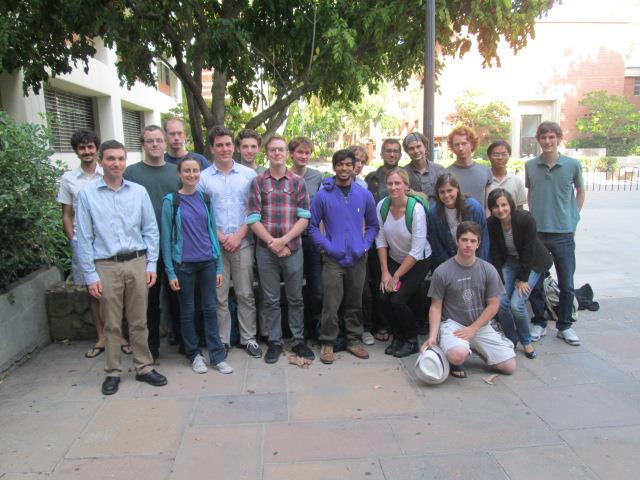UCLA Logic Center# 2013 Undergraduate Summer School

## (This is the 2013 page. Are you looking for the current Summer School?)

The UCLA Logic Center held a three-week summer school for undergraduates in 2013, from Sunday July 14 to Saturday August 3. (Summer school flyer, 8.5 x 11 inches, PDF format.)

The goal of the Center's summer schools is to introduce future mathematicians to central results and techniques from mathematical logic. Courses are very intensive, and reach advanced graduate level material. They are designed to not require specific background in logic, but they do require high mathematical sophistication, for example from upper division or graduate courses in analysis or algebra. The summer school courses serve as good introduction to the kind of research work that students of mathematics can expect in graduate school.

Each course in the summer school met daily for two hours of lecture, and one hour of guided problem solving in small groups. Students each took two of the three courses offered. In addition to the six daily hours of course work (not to mention endless hours of extra work on challenging problem sets over evenings and weekends) there were lectures on topics of current research, social events, and planned outings in the area.

Thanks to an NSF grant (DMS-1044604) and Logic Center support we were able to provide admitted students with a stipend of \$3000, travel allowance up to \$500, and dormitory housing at UCLA for no charge (double occupancy rooms, breakfast and dinner included).### Topics in 2013:

Applied recursion theory
Instructor: Andrew Marks

The precise formalization of computation in the 1930s led to an entirely new perspective with which to approach mathematics: we examine what mathematical objects and transformations can be effectively computed, and for those that can not, the extent to which they fail to be computable. Incomputability results especially are the main focus of recursion theory, with celebrated theorems such as the insolubility of the word problem for groups, and the existence of a PDE with computable initial conditions, but an incomputable solution. Moreover, recursion theory permits a fine analysis of how far from computable a problem is, giving us techniques for establishing what tools can and can not be used to answer particular mathematical questions (as well as providing new methods for solving them). In this course, we develop the basics of recursion theory and then use it to examine a variety of problems from diverse areas of mathematics.

Descriptive set theory
Instructor: Anush Tserunyan

Mathematicians in the early 20th century discovered that the Axiom of Choice implied the existence of pathological subsets of the real line lacking desirable regularity properties (for example nonmeasurable sets). This gave rise to Descriptive Set Theory (DST), a systematic study of classes of sets where these pathologies can be avoided, including, in particular, the definable sets. In the first half of the course, we will use techniques from analysis and set theory, as well as infinite games, to study definable sets of reals and their regularity properties, such as the perfect set property (a strong form of the continuum hypothesis), the Baire property, and measurability.

DST has found applications in dynamical systems, functional analysis, and various other areas of mathematics. Many of the recent applications are via the theory of definable equivalence relations, which provides a framework for studying very general types of classification problems in mathematics. The second half of this course will give an introduction to this theory, culminating in a proof of a famous dichotomy theorem, which exhibits a minimum element among all problems that do not admit concrete classification.

Forcing and independence in set theory
Instructor: Spencer Unger

Set-theoretic forcing is a technique originally introduced by Paul Cohen to prove that the axiom of choice and the continuum hypothesis are independent of the classical axioms of mathematics. Since then it has led to an explosive growth in research, and has been used by set theorists to prove literally hundreds of independence results. In this course we will introduce students to the forcing technique by first studying some of the combinatorial consequences of Martin's Axiom, an axiom which when added to ZFC makes it possible to prove consistency results by means very similar to those used in forcing. We will then introduce forcing itself and show how it can be applied to settle the independence of the continuum hypothesis.